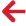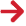﻿ PipeLay > Excel Add-In > Function Reference > Statistics Functions > PLGetNaiveStandardDeviation

# PLGetNaiveStandardDeviation

Navigation:  PipeLay > Excel Add-In > Function Reference > Statistics Functions >

# PLGetNaiveStandardDeviation## PURPOSE

Retrieve the Naive Standard Deviation for the specified parameters in the specified time range from the database.

## HOW IS THIS CALCULATED?

The Naive Standard Deviation is a method used to calculate standard deviation using only a single pass. It is calculated as follows:

Count the number of items in the specified range, n

Sum the values of each item in the range, Sum

Sum the square of each item in the range, SumSq

Calculate the variance, var = ( SumSq - ( Sum2 ) / n) / n

The Standard Deviation is calculated as √ var

This function will return a valid result when n > 1.

## SYNTAX

PLGetNaiveStandardDeviation( DatabaseName, StartTimeIndex, EndTimeIndex, ParameterType, ItemIndex, DOFNo )

## PARAMETERS

 DatabaseName (Character String) The full path and filename to the relevant database. The file extension is optional. StartTimeIndex (Integer) The start time index, from 1 to the number of solutions in the database. EndTimeIndex (Integer) The end time index, from 1 to the number of solutions in the database. This should be a number higher than StartTimeIndex. ParameterType (Integer) The type of parameter to retrieve data for. See Parameter Types for a complete list. ItemIndex (Integer) The index of the required item. This is parameter dependent e.g. for kinematic parameters, this is a node number. DOFNo (Integer) The DOF or Local node number. This is parameter dependent so the ranges should correspond to what is valid for the parameter.

## OUTPUT

A numerical value containing the standard deviation for the specified parameters or one of the following error codes:

 -999999998 Invalid Database. -999999996 Invalid Parameter Number. -999999995 Invalid Time Index. This may be either the start or end time. -999999990 No Data Available. -999999988 Item Index is out of range. -999999985 Local Node Number or DOF is out of range. -999999980 General Read Error. -999999979 PLGetNaiveStandardDeviation function failed. Use the PLGetStandardDeviation function instead.

Error Codes

Parameters

PLGetEnvelopeData

PLGetMaximumValue

PLGetMeanValue

PLGetMedian

PLGetMinimumValue

PLGetMode

PLGetRangeValue

PLGetStandardDeviation